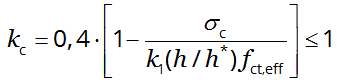For full functionality of this site it is necessary to enable JavaScript. Here are the instructions how to enable JavaScript in your web browser.

# Crack control: the minimum reinforcement area As,min

## Eurocode 2 part 1-1: Design of concrete structures \$(document).ready(function () { var freeExample = \$("#freeExample").length; \$("#mainMenu li#nav-appCate, #mainMenu li#nav-appEC211").addClass("active"); var proUser = false === true || false === true || freeExample !== 0; if (!proUser) { \$('#Input input').keyup(function(){ \$('#AlertSubscribe').modal(); }); \$('#Input select').change(function(){ \$('#AlertSubscribe').modal(); }); }; var tempInput = \$("#tempInput").text(); if (tempInput != "") { \$("#Input").parent("form").deserialize(tempInput); if (proUser) { \$("#acceptBtn").trigger("click"); } }; });  7.3.2 (2)

If crack control is required, a minimum amount of bonded reinforcement is required to control cracking in areas where tension is expected. The required minimum areas of reinforcement may be calculated as follows:

 As,min σs = kc k fct,eff Act (7.1)

where:

As,min
is the minimum area of the reinforcing steel within the tensile zone
Act
is the area of concrete within tensile zone.
σs
is the absolute value of the maximum stress permitted in the reinforcement immediately after formation of the crack. This may be taken as the yield strength of the reinforcement, fyk. A lower value may, however, be needed to satisfy the crack width limits according to the maximum bar size or spacing (cf. 7.3.3 (2))
fct,eff
is the mean value of the tensile strength of the concrete effective at the time when the cracks may first be expected to occur:
fct,eff = fctm or lower, (fctm(t)), if cracking is expected earlier than 28 days
k
is the coefficient which allows for the effect of non-uniform self-equilibrating stresses, which lead to a reduction of restraint forces
= 1,0 for webs with h ≤ 300 mm or flanges with widths less than 300 mm,
= 0,65 for webs with h ≥ 800 mm or flanges with widths greater than 800 mm,
intermediate values may be interpolated
kc
is a coefficient which takes account of the stress distribution within the section immediately prior to cracking and of the change of the lever arm:
* For pure tension kc = 1,0;
* For bending or bending combined with axial forces:
- For rectangular sections and webs of box sections and T-sections:(7.2)

where:

σc
is the mean stress of the concrete acting on the part of the section under consideration:
 σc = NEd/(b⋅h) (7.4)

with

NEd
the axial force at the serviceability limit state acting on the part of the cross-section under consideration (compressive force positive). NEd should be determined considering the characteristic values of prestress and axial forces under the relevant combination of actions
b
the width of the section
h
the height of the section
h*
h* = h     for h < 1,0 m
h* = 1,0 m   for h ≥ 1,0 m
k1
is a coefficient considering the effects of axial forces on the stress distribution:
k1 = 1,5     if NEd is a compressive force
k1 = 2h*/(3h)  if NEd is a tensile force
- For flanges of box sections and T-sections:(7.3)

where:

Fcr
is the absolute value of the tensile force within the flange immediately prior to cracking due to the cracking moment calculated with fct,eff.

This application calculates the minimum reinforcement area As,min from your inputs. Intermediate results will also be given.

First, change the following option if necessary:

Input
mm2
MPa
MPa

Output
kc
the minimum reinforcement area As,min
mm2 (7.1)